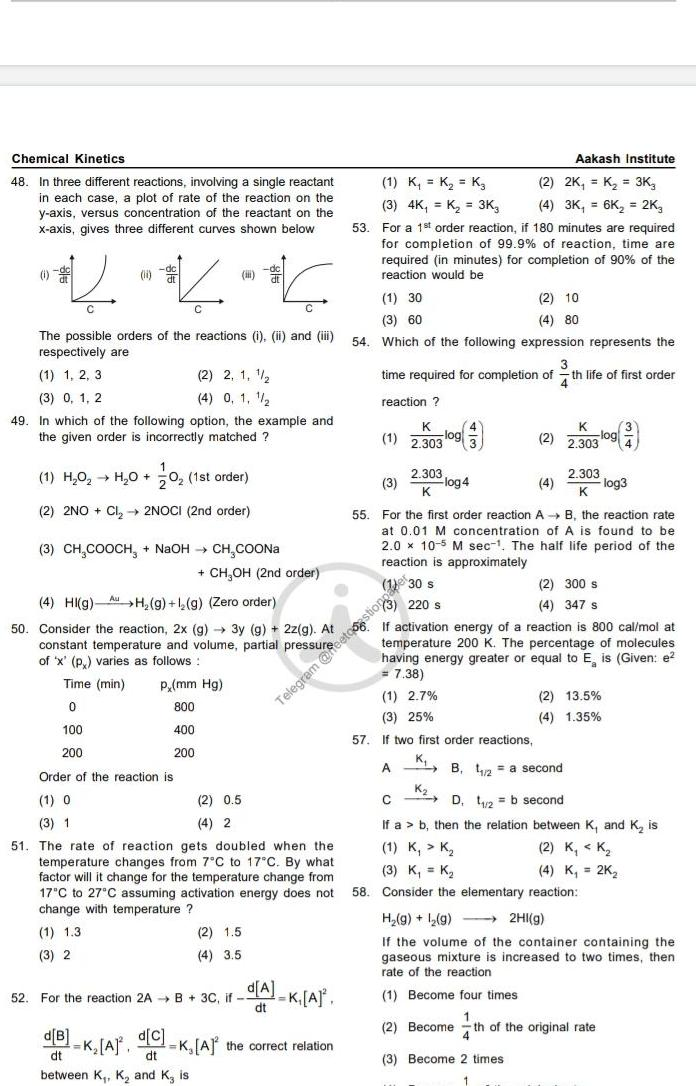Question:

# Chemical Kinetics 48 In three different reactions involving

Last updated: 7/4/2023Chemical Kinetics 48 In three different reactions involving a single reactant in each case a plot of rate of the reaction on the y axis versus concentration of the reactant on the x axis gives three different curves shown below 0 0 The possible orders of the reactions i ii and iii respectively are 1 1 2 3 2 2 1 1 4 0 1 1 2 3 0 1 2 49 In which of the following option the example and the given order is incorrectly matched 1 H O H O 0 1st order 2 2NO Cl 2NOCI 2nd order i 3 CH COOCH NaOH CH COONa K A CH OH 2nd order p mm Hg 800 400 200 d B d C dt dt between K K and K is 2 0 5 4 2 2 1 5 4 3 5 52 For the reaction 2A B 3C if Telegram K A K A the correct relation 4 HI g H g 1 g Zero order 50 Consider the reaction 2x g 3y g 2z g At constant temperature and volume partial pressurece 220 s of x p varies as follows Time min 0 100 200 Order of the reaction is 1 O 3 1 51 The rate of reaction gets doubled when the temperature changes from 7 C to 17 C By what factor will it change for the temperature change from 17 C to 27 C assuming activation energy does not change with temperature 1 1 3 3 2 d A dt 53 Aakash Institute 1 K K K 2 2K K 3K 3 4K K 3K3 4 3K 6K 2K3 For a 1st order reaction if 180 minutes are required for completion of 99 9 of reaction time are required in minutes for completion of 90 of the reaction would be 1 30 2 10 4 80 3 60 54 Which of the following expression represents the time required for completion of th life of first order 4 reaction K 1 2 303 2 303 K 3 log4 55 For the first order reaction A K 2 2 303 1 2 7 3 25 57 If two first order reactions A B the reaction rate at 0 01 M concentration of A is found to be 2 0 x 10 5 M sec The half life period of the reaction is approximately 1 30 s log 2 303 K log3 If activation energy of a reaction is 800 cal mol at temperature 200 K The percentage of molecules having energy greater or equal to E is Given e 7 38 2 300 s 4 347 s KB 2 a second K 2 13 5 4 1 35 C D 2 b second If a b then the relation between K and K is 1 K K 2 K K 3 K K 4 K 2K 58 Consider the elementary reaction H g 2 g 2HI g If the volume of the container containing the gaseous mixture is increased to two times then rate of the reaction 1 Become four times 2 Become th of the original rate 3 Become 2 times